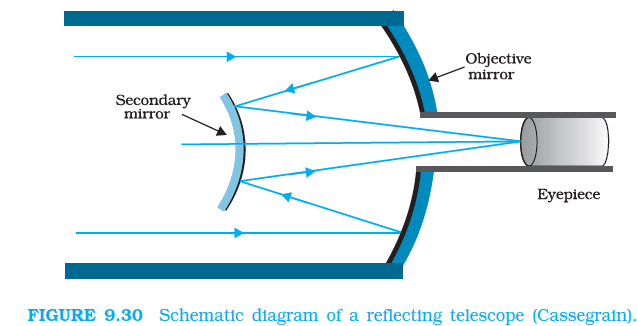# Q9.30  A Cassegrain telescope uses two mirrors as shown in Fig. 9.30. Such a telescope is built with the mirrors 20mm apart. If the radius of curvature of the large mirror is 220mm and the small mirror is 140mm, where will the final image of an object at infinity be?Given,

Distance between the objective mirror and secondary mirror

The radius of curvature of the Objective Mirror

So the focal length of the objective mirror

The radius of curvature of the secondary mirror

so, the focal length of the secondary mirror

The image of an object which is placed at infinity, in the objective mirror, will behave like a virtual object for the secondary mirror.

So, virtual object distance for the secondary mirror

Now, applying  the mirror formula in the secondary mirror:

Exams
Articles
Questions